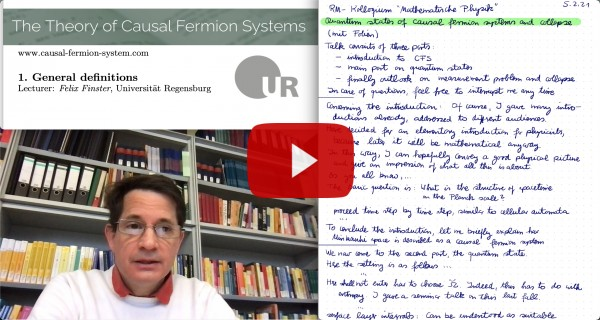# The Theory of Causal Fermion Systems

## The Linearized Field Equations## The Linearized Field Equations

Mimicking the usual procedure for linearizing physical equations, we consider a family $(\tilde{\rho})_\tau$ of measures parametrized by $\tau \in (-\delta, \delta)$ which all satisfy the Euler-Lagrange equations and linearize in the parameter $\tau$. Assuming that the measures are obtained by a push-forward and a change of weight, i.e.

$\tilde{\rho}_\tau = (F_\tau)_* \big( f_\tau \,\rho \big)$

with $F_\tau \in C^\infty(M, \F)$ and $f_\tau \in C^\infty(M, \R^+)$, these variations can be described linearly in $\tau$ by the jet

$\mathfrak{v} := \frac{d}{d\tau} \big( f_\tau, F_\tau \big) \big|_{\tau=0}$

(for details see [jet16]; more general variations allowing for a so-called fragmentation of the measure are considered in [perturb17, Section 5]). The fact that the variation described infinitesimally by the jet preserves the Euler-Lagrange equations gives rise to the linearized field equations

$\la \mathfrak{u}, \Delta \mathfrak{v} \ra|_M = 0 \:,$

to be satisfied for all test jets $\mathfrak{u}$, where the Laplacian $\Delta$ is defined by

$\la \mathfrak{u}, \Delta \mathfrak{v} \ra(x) := \nabla_{\mathfrak{u}} \bigg( \int_M \big( \nabla_{1, \mathfrak{v}} + \nabla_{2, \mathfrak{v}} \big) \L(x,y)\: d\rho(y) – \nabla_\mathfrak{v} \mathfrak{s} \bigg) \:.$

(for details see [jet16] or the summary in [perturb17, Section 3.3]).

## The Surface-Layer One-Form

The linearized field equations gives rise to conservation laws expressed in terms of surface layer integrals, as we now outline. In [osi18] the so-called surface layer one-form is introduced by

$\gamma^\Omega_\rho(\mathfrak{v}) = \int_\Omega d\rho(x) \int_{M \setminus \Omega} \big( \nabla_{1,\mathfrak{v}} – \nabla_{2,\mathfrak{v}} \big) \L(x,y) \Big) \: d\rho(y) \:.$

This surface layer integral is conserved if the jet $\mathfrak{v}=(0,v)$ has a vanishing scalar component. If $v$ generates a symmetry, this conservation law gives back the Noether-like theorems. It generalizes the Noether-like theorems because all we need is that $v$ is a solution of the linearized field equations, but no symmetry assumptions are needed.

## The Symplectic Form

In [jet16] it is shown that the linearized field equations also give rise to a another conservation law. Indeed, for two solutions $\mathfrak{u}$ and $\mathfrak{v}$, the symplectic form $\sigma_\Omega$ defined by

$\sigma^\Omega_\rho(\mathfrak{u}, \mathfrak{v}) = \int_\Omega d\rho(x) \int_{M \setminus \Omega} \Big( \nabla_{1,\mathfrak{u}} \nabla_{2,\mathfrak{v}} \L(x,y) – \nabla_{1,\mathfrak{v}} \nabla_{2,\mathfrak{u}} \L(x,y) \Big) \: d\rho(y)$

is conserved (again in the sense that its value is preserved if $\Omega$ is changed by a compact set).

In [action17] it is shown that in the example of causal fermion systems in Minkowsi space and the Maxwell field, the above surface layer integral reduces to the symplectic form of classical field theory.

## The Surface Layer Inner Product

In [osi18] a general class of conserved surface layer integrals is derived. Most interesting for the applications is the surface layer inner product defined by

$(\mathfrak{u}, \mathfrak{v})^\Omega_\rho = \int_\Omega d\rho(x) \int_{M \setminus \Omega} \Big( \nabla_{1,\mathfrak{u}} \nabla_{1,\mathfrak{v}} \L(x,y) – \nabla_{2,\mathfrak{v}} \nabla_{2,\mathfrak{u}} \L(x,y) \Big) \: d\rho(y)$

As a consequence of the second derivatives acting on the same variable, this surface layer integral depends on the choice of coordinates on $\F$. A related issue is that it is conserved only approximately. In [action17] it is shown that in the example of causal fermion systems in Minkowsi space and the Maxwell field, it reduces to the scalar product used in quantum field theory obtained by inserting a frequency splitting into the symplectic form. The freedom in choosing the frequency splitting corresponds to the freedom in choosing the coordinates of $\F$.

The surface layer inner product is used in the existence theory for the linearized field equations for getting energy estimates (see [linhyp18]). Moreover, it is used in [FK18] for giving the linearized solutions an almost complex structure.